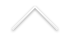Algorithms are mathematical objects. The development of proven efficient algorithms is a challenge that requires tools from several areas of mathematics. Complexity is the study of lower or upper bounds for the efficiency of algorithms for a certain problem. Finding precise lower bounds can be extremely diff cult. We are particularly interested in algorithms and complexity for continuous problems, as such that arise in numerical analysis.

Impact and applications. From a technological viewpoint, a better mathematical understanding of numerical analysis means faster and more reliable algorithms. In particular, we do not have a satisfactory technology to solve systems of polynomial equations. Improvemnents in this technology would be useful for subjects such as mechanical engineering, chemical/biochemical kinetics, computer graphics, nonlinear optimization, control theory, etc...

Connections with other subjects within mathematics. Input and output spaces of numerical problems may be linear spaces or more generally smooth manifolds. One may assume a probability measure in input space and also some group action invariance. Invariants as the condition number can be then interpreted as a random variable, and the probability for a problem to be ill-posed can be estimated. But the condition number can also be related with the reciprocal distance to a discriminant variety, and may be bounded in terms of the arithmetic properties of the discriminant.

Researchers:

• Gregorio Malajovich